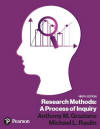﻿ Manual Computation of the MeanGraziano & Raulin
Research Methods (9th edition)

## Computational Procedures for the Mean

To compute the mean of any group of scores, simply add up the scores and divide by the number of scores.

For example, if we have 5 scores (35, 24, 43, 32, 37), the mean would be the sum of those scores (171) divided by the number of scores (5), which is 34.2. The formula for computing the mean is:The symbol for the mean is an X with a bar over it (read X bar). The numerator of the formula is summation notation (read Sigma X). It simply means to sum all of the X scores. The denominator (N) is the number of scores. We will be using similar notation throughout this section on manual computation of statistics.

There is a more detailed section on statistical notation included elsewhere on this website.